# Solve Equations with Variables on Both Sides

 Would You Rather Listen to the Lesson?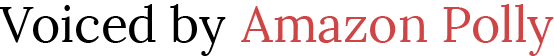## Initial Activity: “Which is heavier? A kilogram of nails or a kilogram of salt?”

And that’s just the perfect introduction when I teach how to solve equations with variables on both sides.  Usually, my students are just getting good at solving one and two-step equations, and then… they are faced with variables on both sides!  It can be confusing at first.  They wonder about when to divide the coefficient, what to do with the variables, how to move the terms around.

Prepare the following materials and keep these hidden. Just let them out at the right time.

1. Nails (pre-weighed and each are placed inside a clear/transparent zip lock, with weights labeled)
• 1 kilogram
•kilogram
•kilogram
1. Salt (pre-weighed and each are placed inside a clear/transparent zip lock, with weights labeled)
• 1 kilogram
•kilogram
•kilogram
1. 2 Weighing Scales (Digital or Analog – whichever is available)

I always bring NAILS and SALT and let my students decide which of the two is heavier/lighter as I let them hold these materials by hand. I pick five students (I may add some more) and ask each one which is heavier/lighter. This initial activity is a good start as I let my students justify their answers.

This is a perfect time to confirm their answers by taking out the weighing scales. Don’t forget to emphasize the goal of this activity is to keep the weight of the nails and salt the same at all times!

• I start with 1 kilo of NAILS and 1 kilo of SALT.
• I place a kilo of nails on one scale and a kilo of salt on the other.
• I then ask them the same question I asked when the class started: “Which is heavier? A kilogram of nails or a kilogram of salt?”
• I always like seeing their reactions as they confirm that both materials exactly weigh the same.
• I always emphasize that heavier or lighter things/materials weigh exactly the same if they have the same weight.
• Then, I add half more kilo of nails.
• Again, I ask them the same question I did when the class started: “Which is heavier? A kilogram of nails or a kilogram of salt?”
• The answer is so obvious that I expect my students to say that this time, the NAILS are heavier than the SALT.
• Ask: “How heavy are the nails now?
• I expect that my students would say that the nails are a kilo and a half heavier than the salt.
• I then give a follow up question: “What are we going to do keep the weight of the NAILS and SALT the same?”
• Some students may answer: “ADD half more kilo of salt to keep the weights the same.
• Confirm the answer by asking a student to add half a kilo of salt to the scale.
• Emphasize that to always keep the weight of the NAILS and the SALT the same, the same amount of weight must be added to each scale..
• You can play around with the materials you have so long as the idea of having both the nails and the salt weigh the same, and how it is done, is clear.
• Will this also work for subtraction?
• Now, placekilo of nails on one scale andkilo of salt on the other.
• It is obvious that the weight of the nails and the salt are the same.
• I can ask a student to take awaykilo of nails from the scale leaving it withkilo of nails whilekilo of salt remains the same.
• I then throw the question: “Which is heavier?kilogram of salt orkilogram of nails?
• The answer is so obvious that I expect my students to say that this time, the SALT is heavier than the NAILS.
• Ask: “Without using addition, what are we going to do keep the weight of the NAILS and SALT the same?”
• Some students may answer: “Also TAKE AWAY a quarter of a kilo of salt to keep the weights the same.
• Confirm the answer by asking a student to take away a quarter of a kilo of salt from the scale.
• By this time, they already have an idea that to keep the weight of the nails and the salt the same without using addition, the same amount of weight must be taken away from both scales.

When I introduce Solving One-Step Equations and Two-Step Equations, I always start off by helping the students understand the goal: to isolate the variable. The initial activity is my springboard in making my students understand how to isolate the variables in Solving Equations with Variables on Both Sides using the Properties of Equality. By this time, students have a clearer picture what balance means.

Going back to the NAIL and SALT (if they have equal initial weight), adding the same amount of weight to each of them does not change the fact that they are still EQUAL. The same thing goes for subtraction.

In solving equations, to isolate the variable, students must take away the other terms from one side of the equation to the other, vice versa, while keeping the equation balanced. “Whatever you do to one side, you must do to the other!” The next activity will make it a bit easier for students to understand how the entire process is done since they already have an idea how to Solve One-Step and Two-Step Equations.

As much as I don’t want my students to just let memorization do its thing, the emphasis of using the different properties of equality is of HUGE importance. This guides the students to keep the equation balanced. This makes them realize that we are not merely “moving sides” and “changing signs”, It is actually the Properties of Equality that does the magic!

I always ask them to remember the phrase:

An equation can be solved correctly if and only if THE BALANCE IS RIGHT!

In Solving Equations with Variables on Both Sides, the goal is to:

GET ALL THE VARIABLES ON THE SAME SIDE

GET ALL THE CONSTANTS ON THE SAME SIDE

Students start to notice that when they move terms from one side of the equal sign to the other, they just change signs.  A positive x becomes a negative x on the other side of an equation.  A negative 3x becomes a positive 3x when it moves to the other side.  But never forget to tell them that the Properties of Equality does the magic!

## Set up for the Lesson: Solve Equations with Variables on Both Sides

1. Give every student a Balance Scale Template.
2. Provide post-it notes or small pieces of paper for the equations and terms.
3. Have students work in pairs or independently.
4. Provide each student with the Solve Equations with Variables on Both Sides Worksheet.

## Launch the Lesson: Solve Equations with Variables on Both Sides

Tell your students to visualize an equation as a balance scale. A balance scale is an instrument used for weighing with a balance beam and two pans. These two pans will remain in balance if each of its pans contain exactly the same weight. You can always go back to the 1 Kilogram of NAILS and 1 Kilogram of SALT used in the initial activity.

Surely, the balance scale will remain in balance because heavier or lighter things/materials weigh exactly the same if they have the same weight.

Hold up the balance scale template, you may opt to paste it on the board so it’s easier for everyone to see. Using the balance scale, help your students visualize the equation:

3x + 1 = x + 9

• Use Post-Its to represent the variables and constants.
• You may use different colored Post-Its to distinguish a variable from a constant,
• Just make sure that all variables have same colors, the same goes for the constant.
• Use may use Orange Post-Its to represent the variables.
• Use may use Pink Post-Its to represent the constants.
• Arrange the given equation on top of the “Balance Scale Template”, making sure that:
• the left side of the equation is aligned with the left pan of the balance scale
• the equal sign (=) is aligned with the beam
• the right side of the equation is aligned with the right pan of the balance scale

3x + 1                     =                     x + 9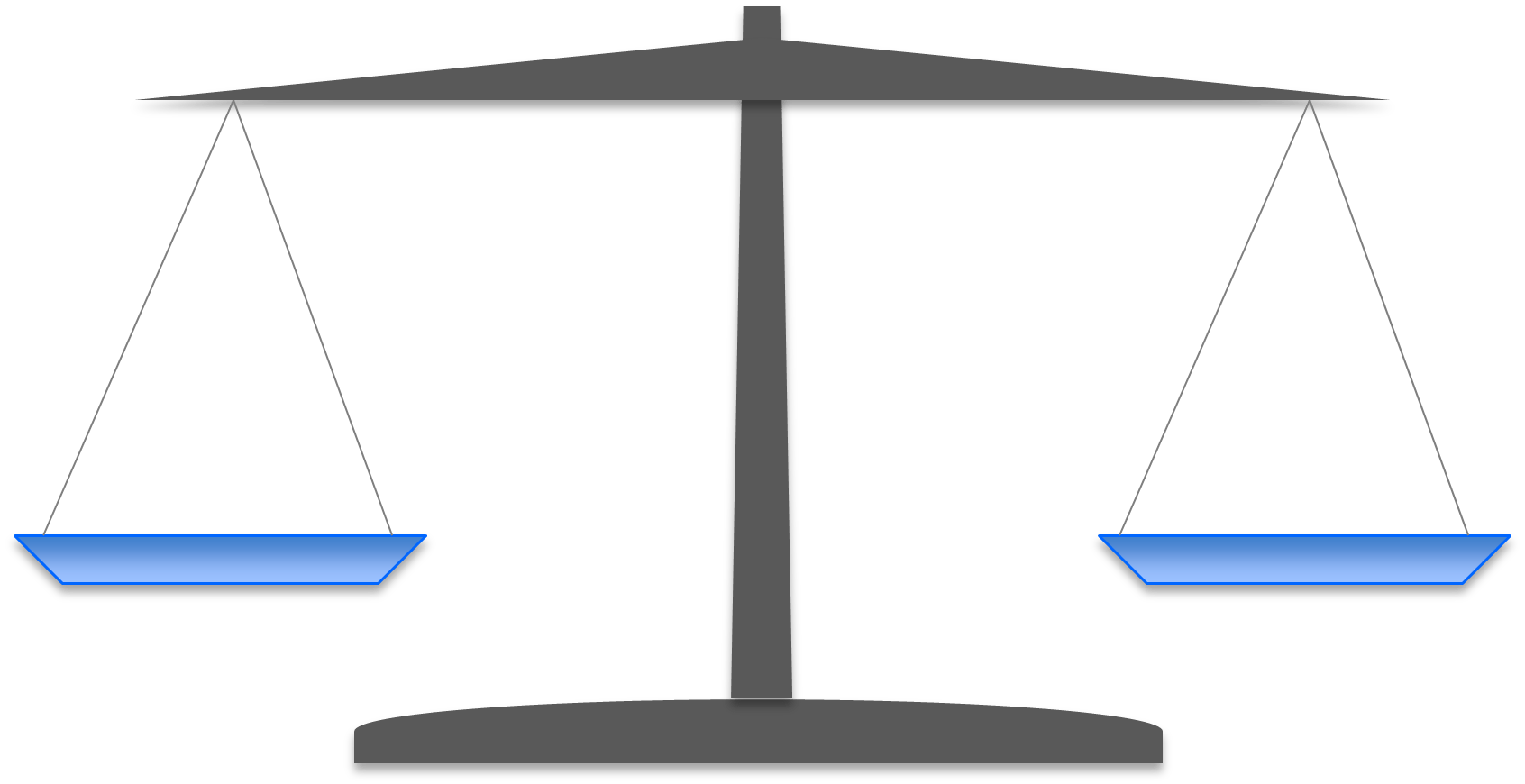• Use a marker to label each ORANGE Post-Its with the variable x. (Don’t forget to carry the sign.)
• The PINK Post-Its represent the constants in the given equation. For example, if the constant is 9 then there should be 9 PINK Post-Its
• On the left pan, place 3 ORANGE Post-Its, each labeled with x, and one PINK Post-It labeled with because the constant is 1.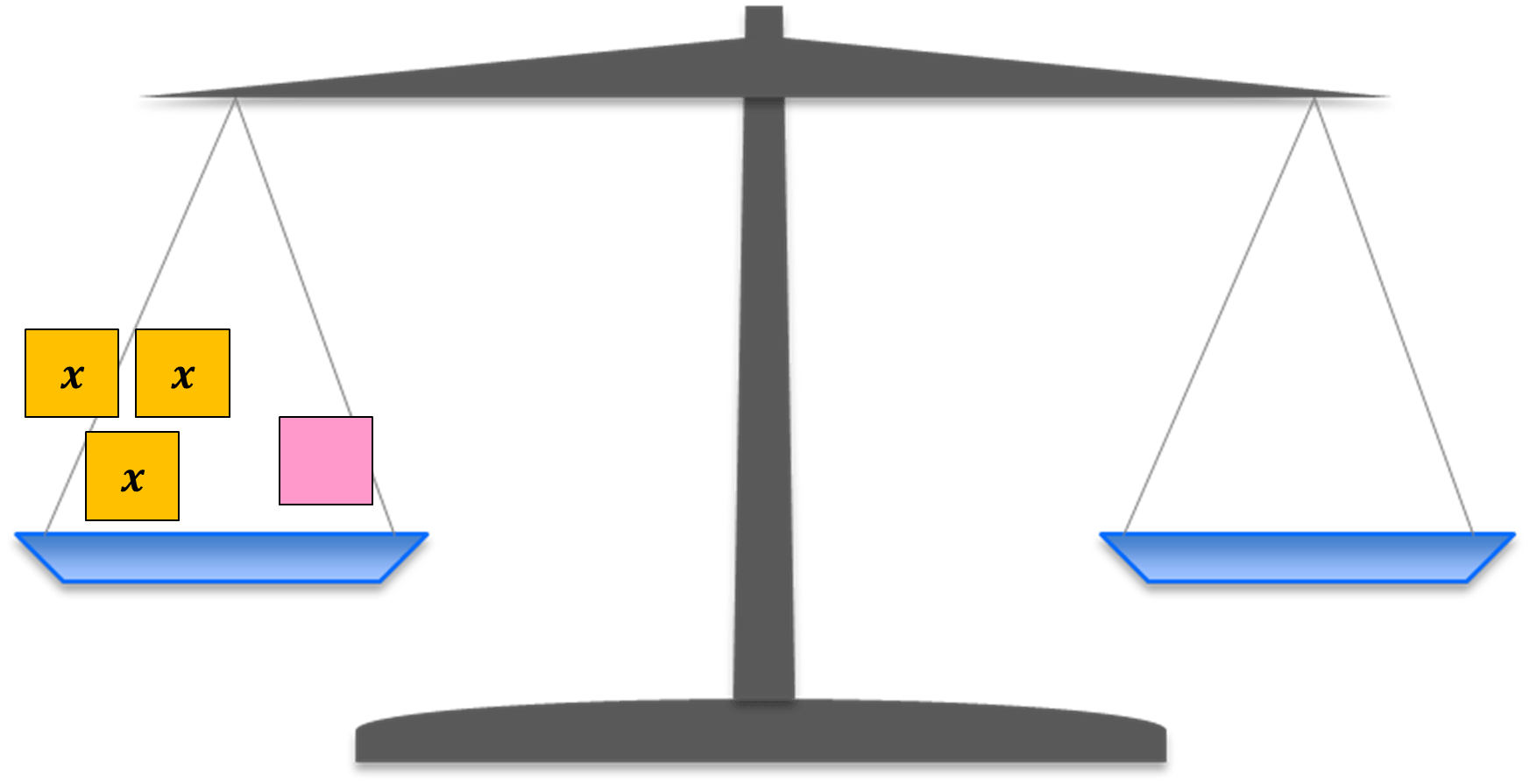• On the left pan, place 1 ORANGE Post-Its, labeled with x, and nine PINK Post-It because the constant is 9.• Emphasize that to keep the balance scale “balanced” whatever is done to the left pan, the same must be done to the right. Even though we do not know the value of x, both pans weigh exactly the same amount.
• Emphasize that when we remove one ORANGE post-it from each pan, the pans will still be in balance.• This means that when we subtract x on both sides of an equation, both sides will remain equal.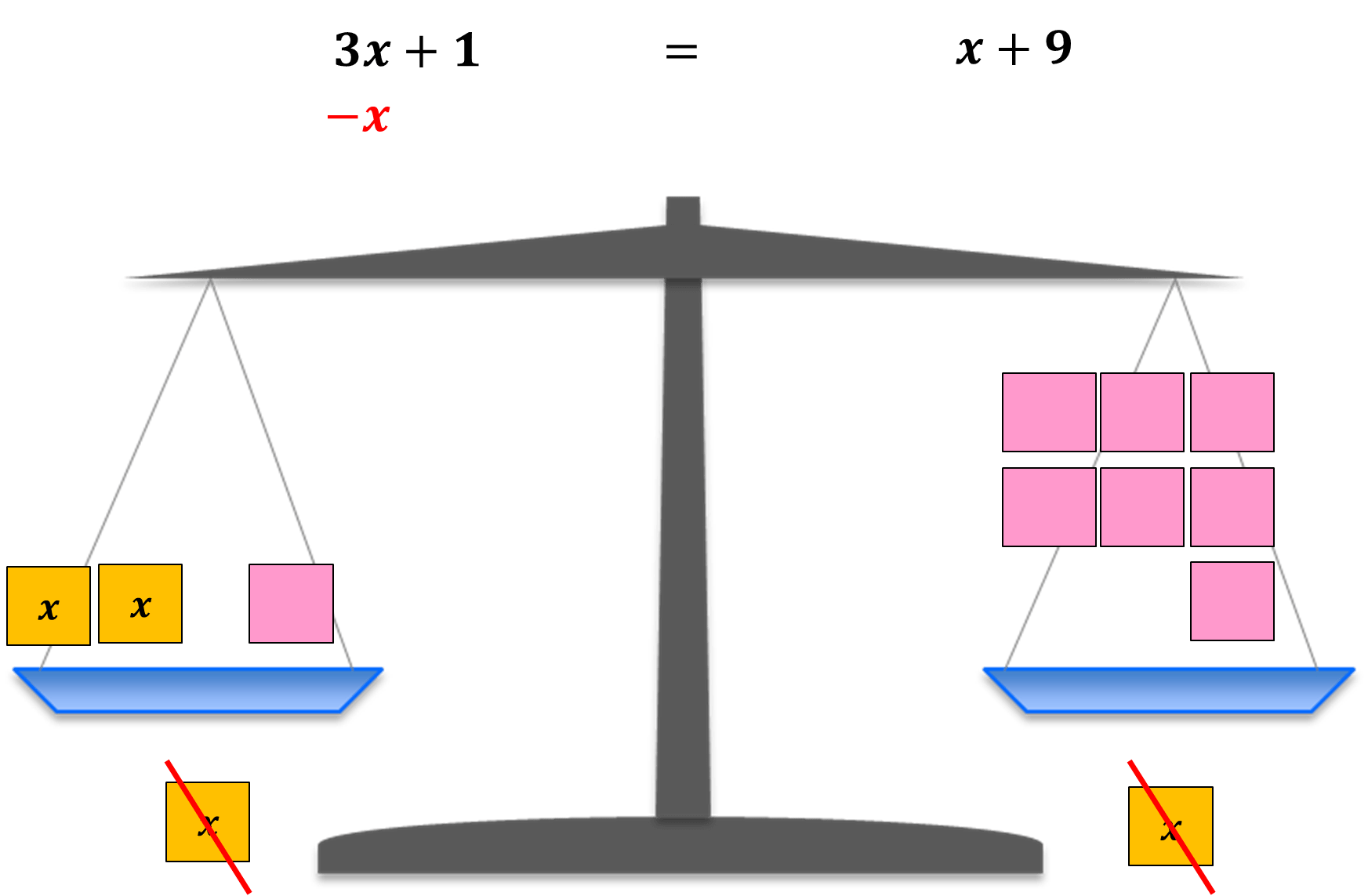• The students than will get a clearer picture of how the Properties of Equality work and how it helps achieve the goal:

Keep all variables on the same side

• Using the illustration above, emphasize that to move all variables to the left side of the equation, we need to subtract x to both sides of the equation.

3x – x + 1 = x – x + 9

3x – x + 1 = 9

2x + 1 = 9

• At this point, students may be familiar with the equation 2x + 1 = 9. This equation involves “Solving Two-Step Equations”. You may have students who know exactly what to do to solve the equation, but for the benefit of those who are still confused, the balance scale is a good guide.
• Now that we have kept all variables to the left side of the equation, it’s time move all constants on the same side as well.

Move all constants to the right side of the equation• Using the illustration above, emphasize that to move all constants to the right side of the equation, we need to subtract to both sides of the equation.

2x + 1 – 1 = 9 – 1

2x = 8

• Now the equation can be solved by dividing both sides of the equation by 2.• Let the students check if the solution is correct by reminding them to substitute the value of to the original equation.

3x + 1 = x + 9, x = 4

3(4) + 1 = 4 + 9

12 + 1 = 4 + 9

13 = 13

• By this time, the concept of the Properties of Equality used in Solving Equations with Variables on Both Sides was clearly explained using a Balance Scale. Remind them of these important notes:
• A balance scale is a good representation of the concept of equations.
• Whatever you do to one side of an equation, the same must be done to the other side to keep the balance and/or equality.
• By applying the properties of equality, move all variables to the same side of the equation (usually to the left).
• By applying the properties of equality, move all constants to the same side of the equation (usually to the right).
• If you think the students have a good understanding of the concept, it’s time to introduce the easier way to solve equations with variables on both sides by modeling it using a balance scale.

Making things easier…

• Present the equation: 5x – 6 = -4x + 3
• Instruct the students to get their ORANGE and PINK Post-Its.
• Write each term with variables on an ORANGE Post It. Don’t forget to carry the sign.• Flip over the ORANGE Post Its and write down the term, but this time with its opposite sign.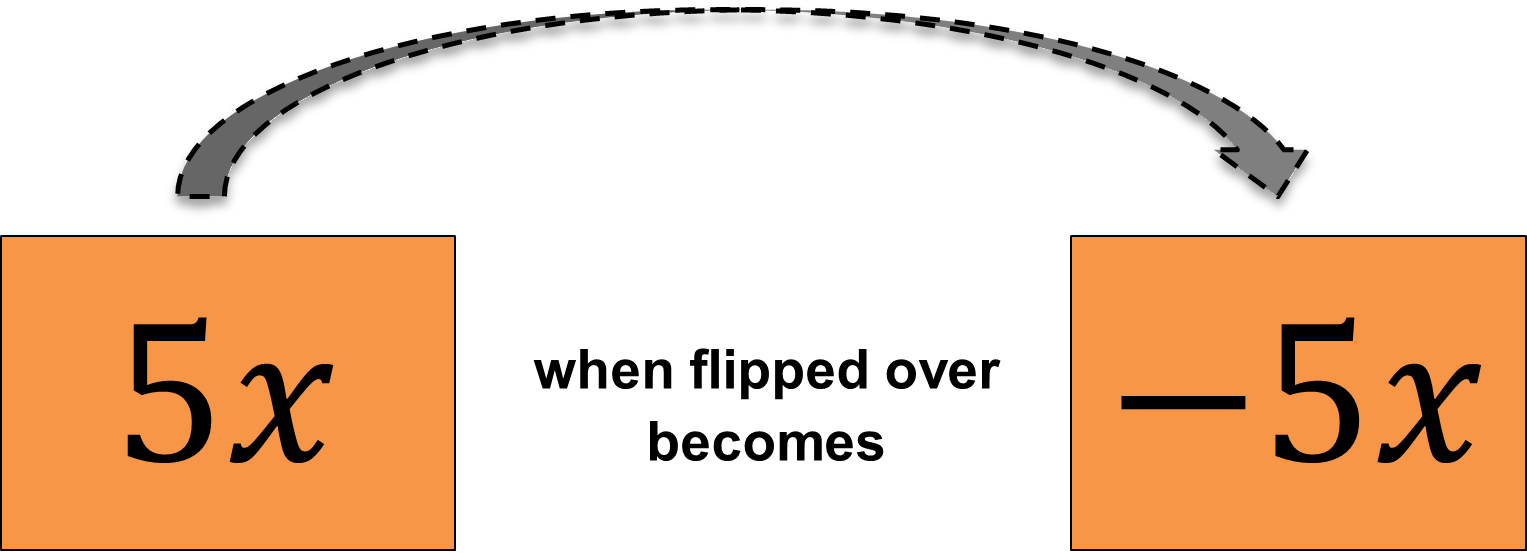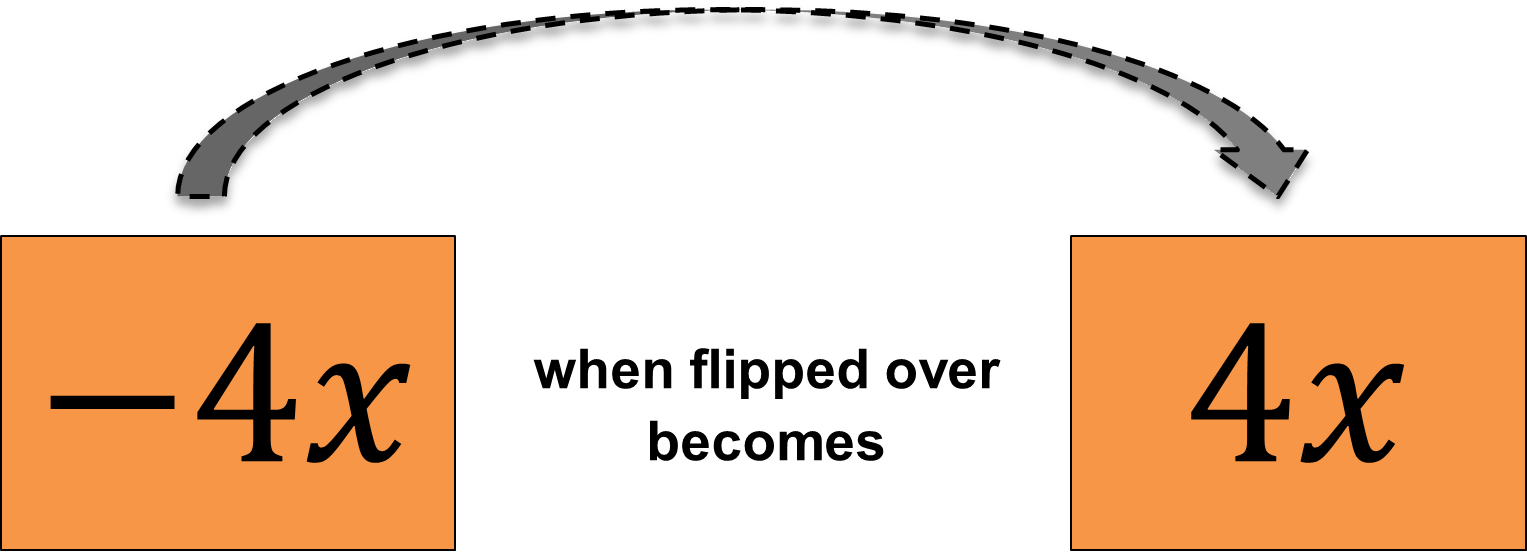• Write each constant on a PINK Post It. Don’t forget to carry the sign.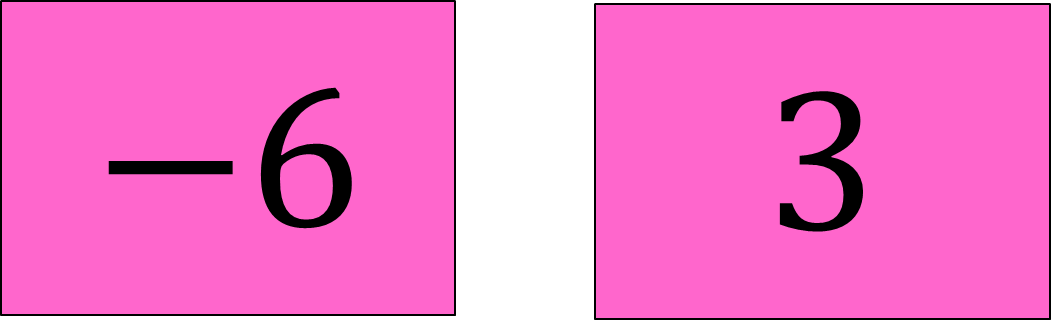• Flip over the PINK Post Its and write down the constant, but this time with its opposite sign.• Go back to the balance scale template on the board. Write down the given expression on top of the balance scale.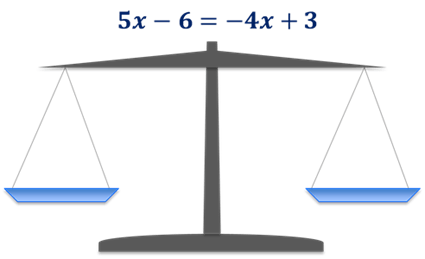• The expression on the left side goes to the left pan and the one on the right goes to the right pan.• Place the post its at the correct pan.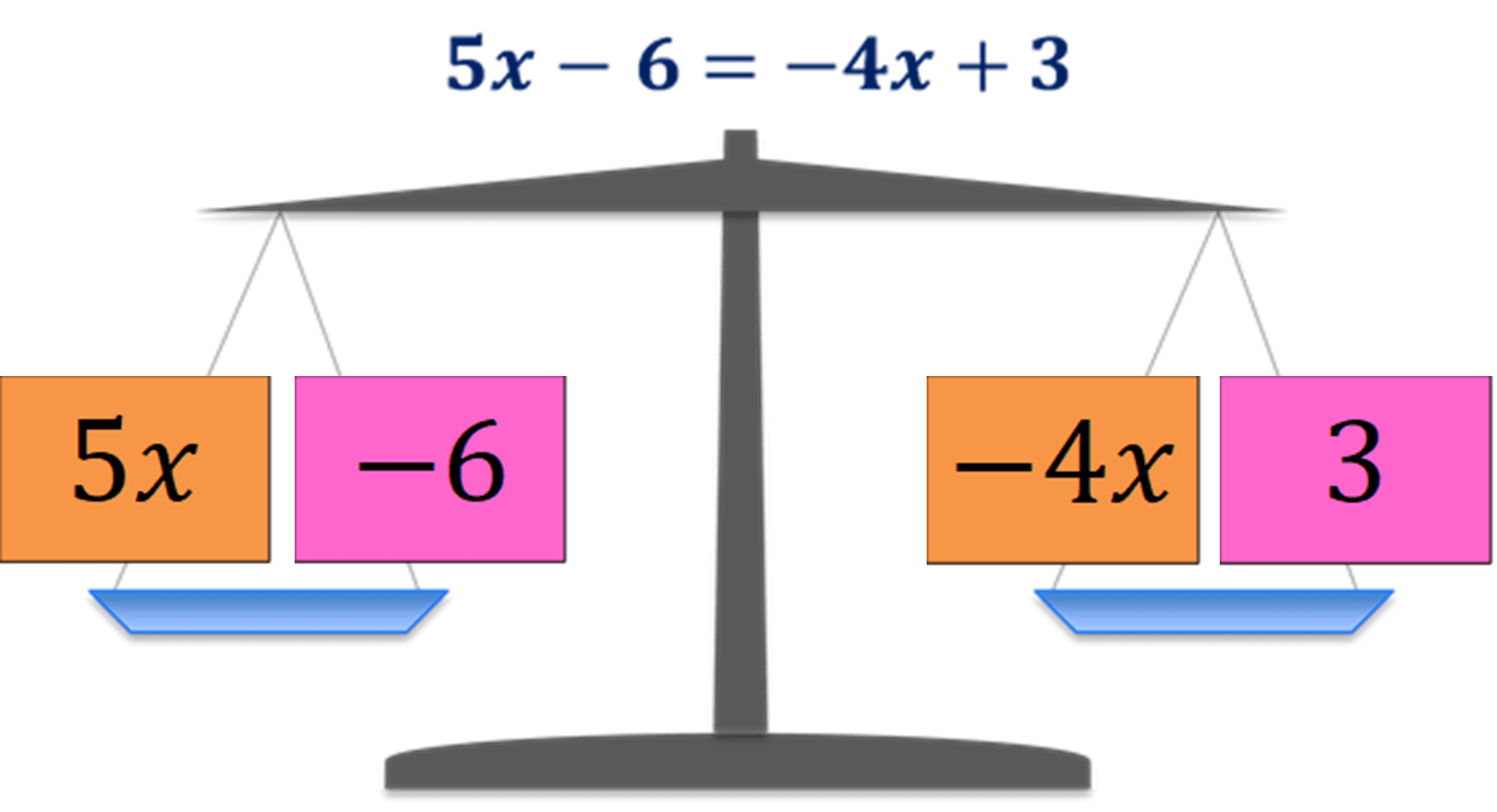• Remind the students of the goal.
• Move all variables to the same side (usually at the left side of the equation)
• Move all constants to the same side (usually at the right side of the equation)
• Now let’s start to move the variables to where they must be.
• To move the variables to the left pan, the sign of the term must change to its opposite sign. To do this, just flip over the post it.• -4x becomes 4x when moved to the left pan.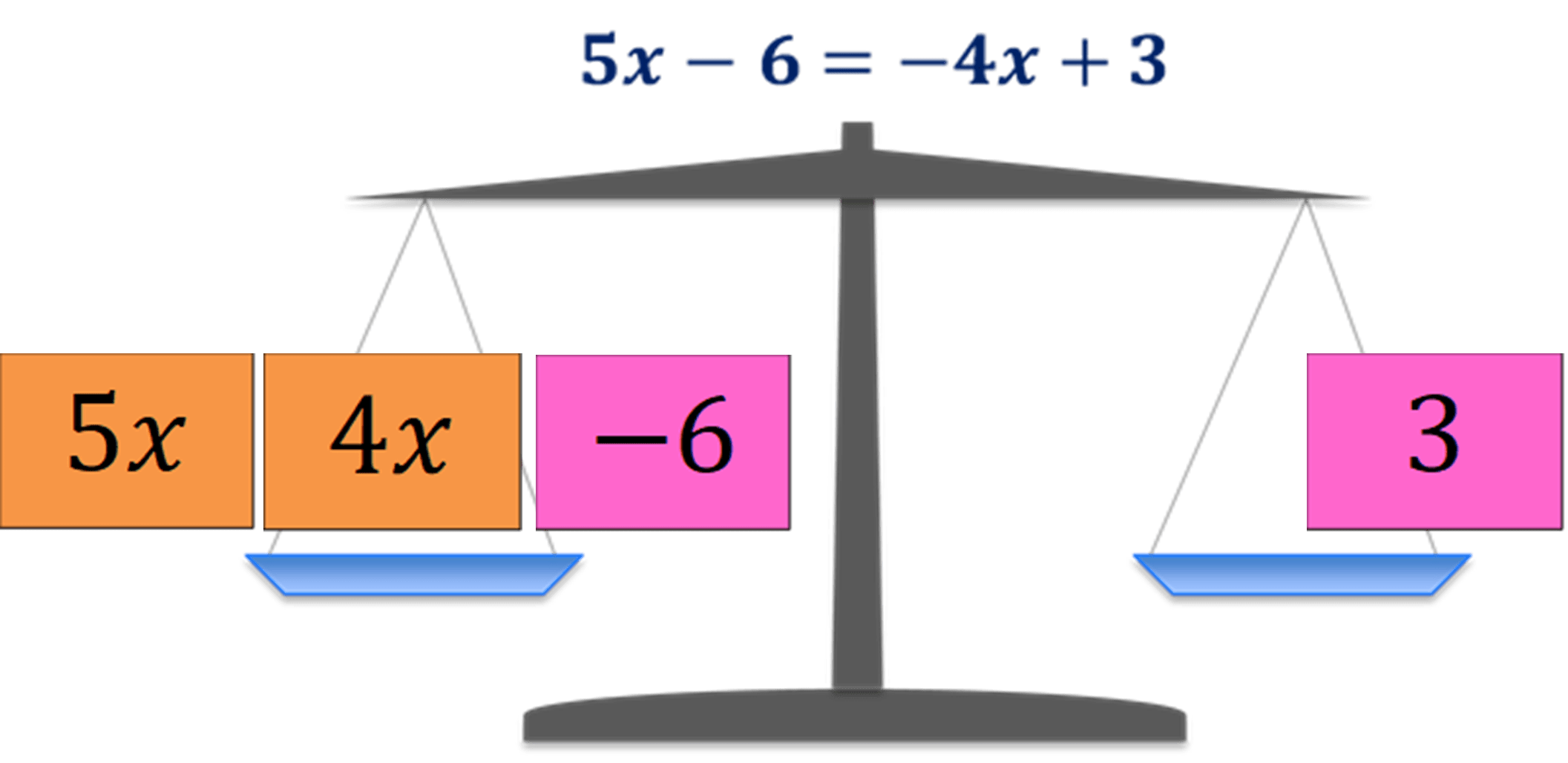• Now let’s move the constant to where they must be.
• To move the constants to the right pan, the sign of the constant must change to its opposite sign. To do this, just flip over the post it.• Now, the equation doesn’t look messy anymore and it’s easier to solve the equation.

5x + 4x = 6 + 3

9x = 9

Divide both sides by 9.• Always check if the solution is correct by replacing the value of in the original equation.

5x – 6 = -4x + 3, x = 1

5(1) – 6 = -4(1) + 3

5 – 6 = -4 + 3

-1 = – 1

Therefore, the solution of the equation 5x – 6 = -4x + 3 is 1.

• After you demonstrate on the board, you can ask if anyone has any questions, and then tell your class that they will be solving equations with variables on both sides.
• Remind them of the following:
• Do not to forget to flip the post its if they move it from the left pan to the right, and vice versa.
• Do not to forget the goal, to isolate the variable on one side and the constant to the other side.
• Show the steps when they solve for the unknown.

Solve Equations with Variables on Both Sides Activity: If the Balance is Right!

After showing the first example on how to Solve Equations with Variables on Both Sides using the “Balance Scale Template”. Its time to introduce the activity: If the Balance is Right!

• Remind them that in moving a variable or a constant from one pan to the other, the sign changes.
• If we have 2x on one pan, moving it to the other pan will make it -2x by flipping the post-it over.
• Everyone should have a balance scale template, with blank pans on both sides.
• Students will determine which side they move the variables and which side they move the constants.
• Use post it notes or cards. A great way for this to run smoothly is to have the students write the opposite value on the opposite side of each post it.  (Or if you are just providing equations, the students should make their own cards first, then flip them over and write the opposite of each.)
• For example:
• If there’s a post it that says 2x, students can flip it over and write -2x.
• If there’s a post it that says -5x then students flip it over and write 5x.
• This is also true for the constant too, -3 when flipped is 3  etc…
• Use may use Orange Post-Its to represent the variables.
• Use may use Pink Post-Its to represent the constants
• Students should:
• Find a pair.
• Choose an equation.
• Model each equation on the balance scale template.
• Move the variables to one side and constants to the other (don’t forget to flip to change the sign!)
• Record the steps and solve the equation
• Check it! Plug back in for x and check to see if you’re right

## Reflecting on the Activity

• Talk about the patterns students saw and the equations that were most difficult. Have the students share some of their work and equations with each other and the class.
• Ask if anyone moved the variables and discovered that the variable term was now negative. This is still solvable, but often if students can try and move the variables so that they end up positive, the next steps are easier.
• Here’s an example to work through together.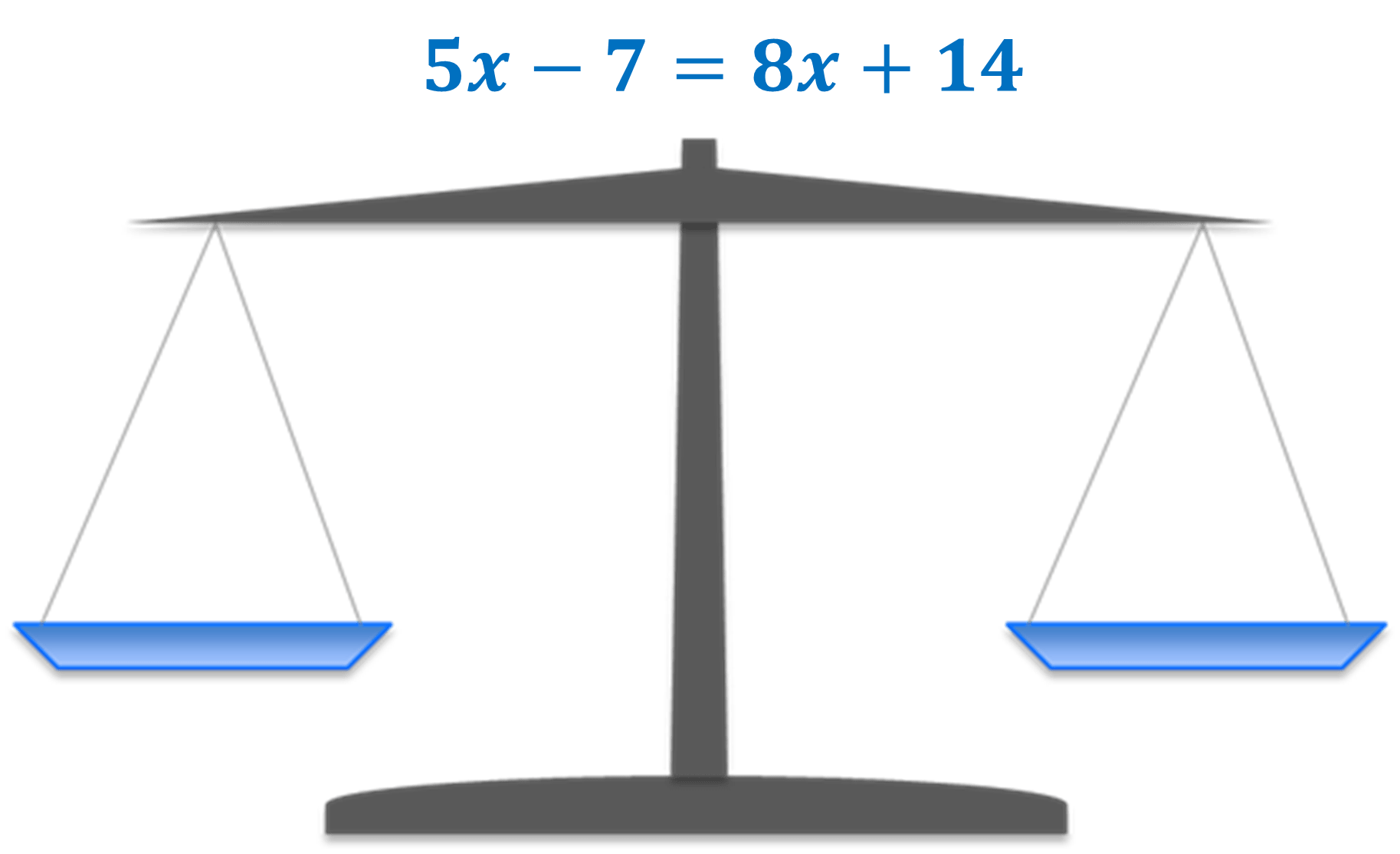• Get ready with the post its. Using ORANGE for the variables and PINK for the constants.
• Write the variable on the orange post its. Flip the post it and don’t forget to write its opposite value of the term.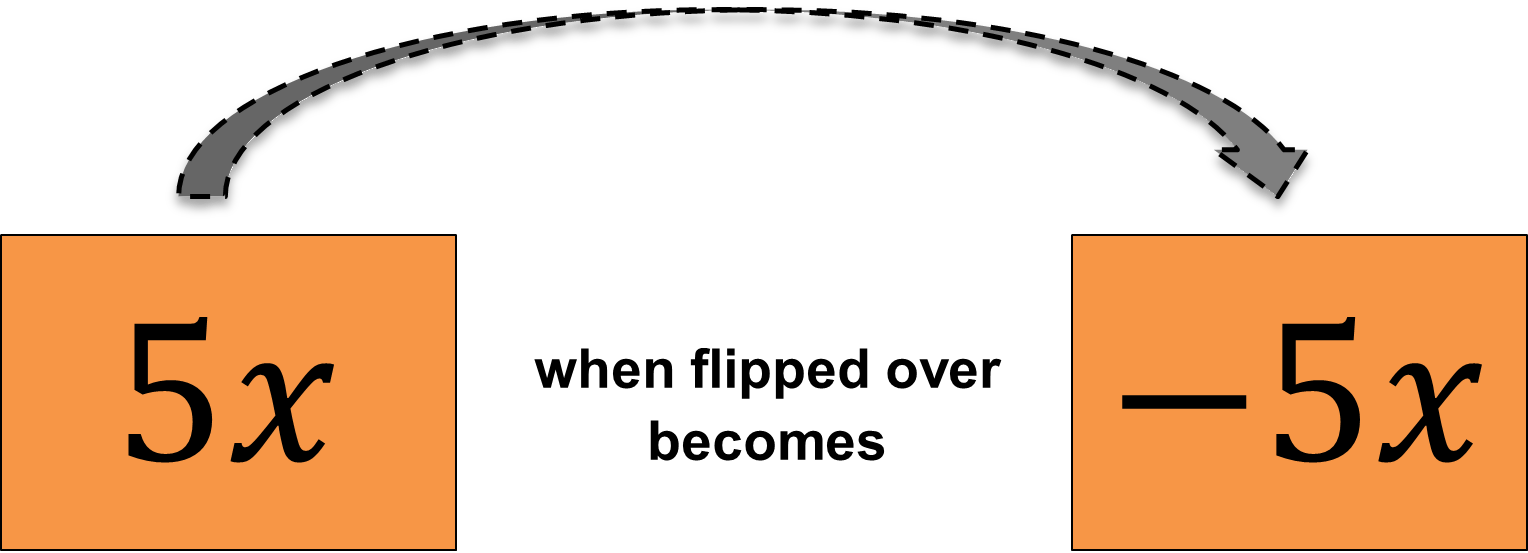• Do the same for the constants.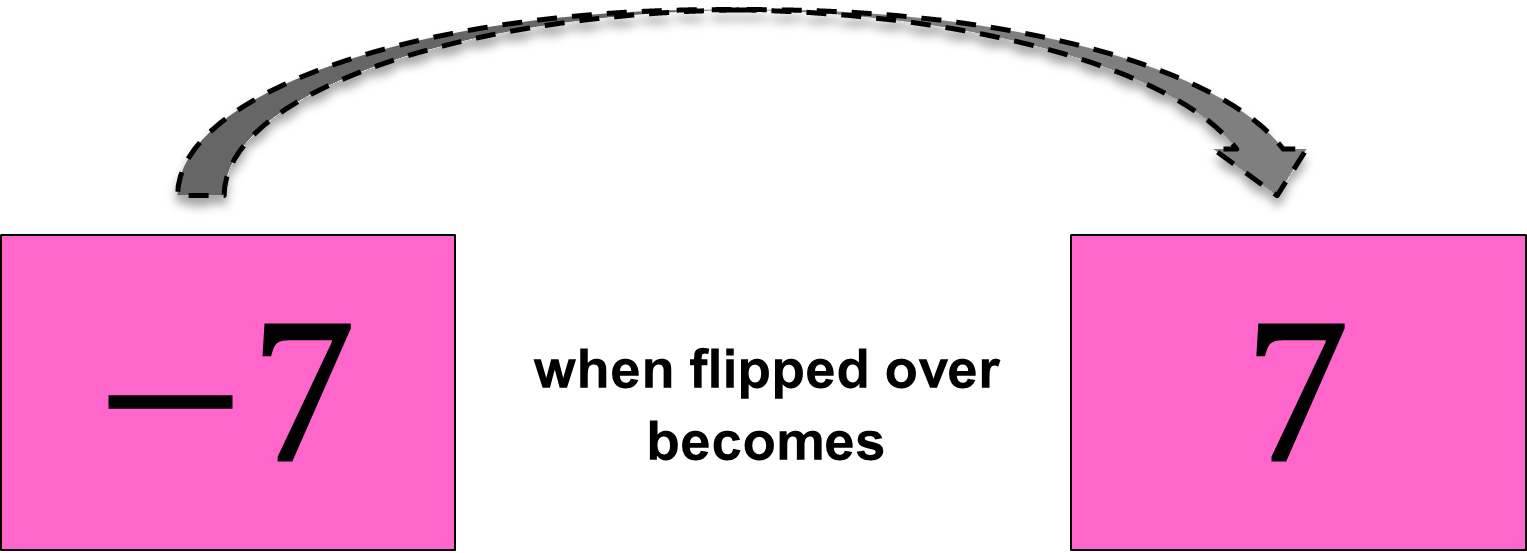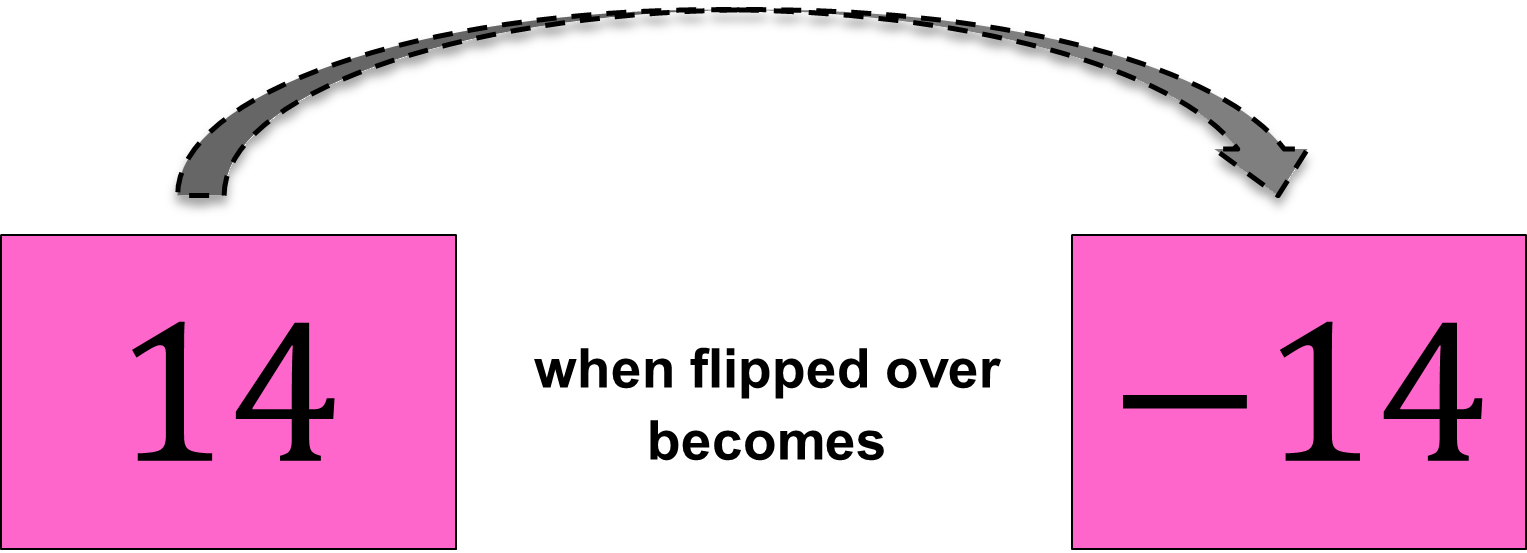• Position the post its at the appropriate pan.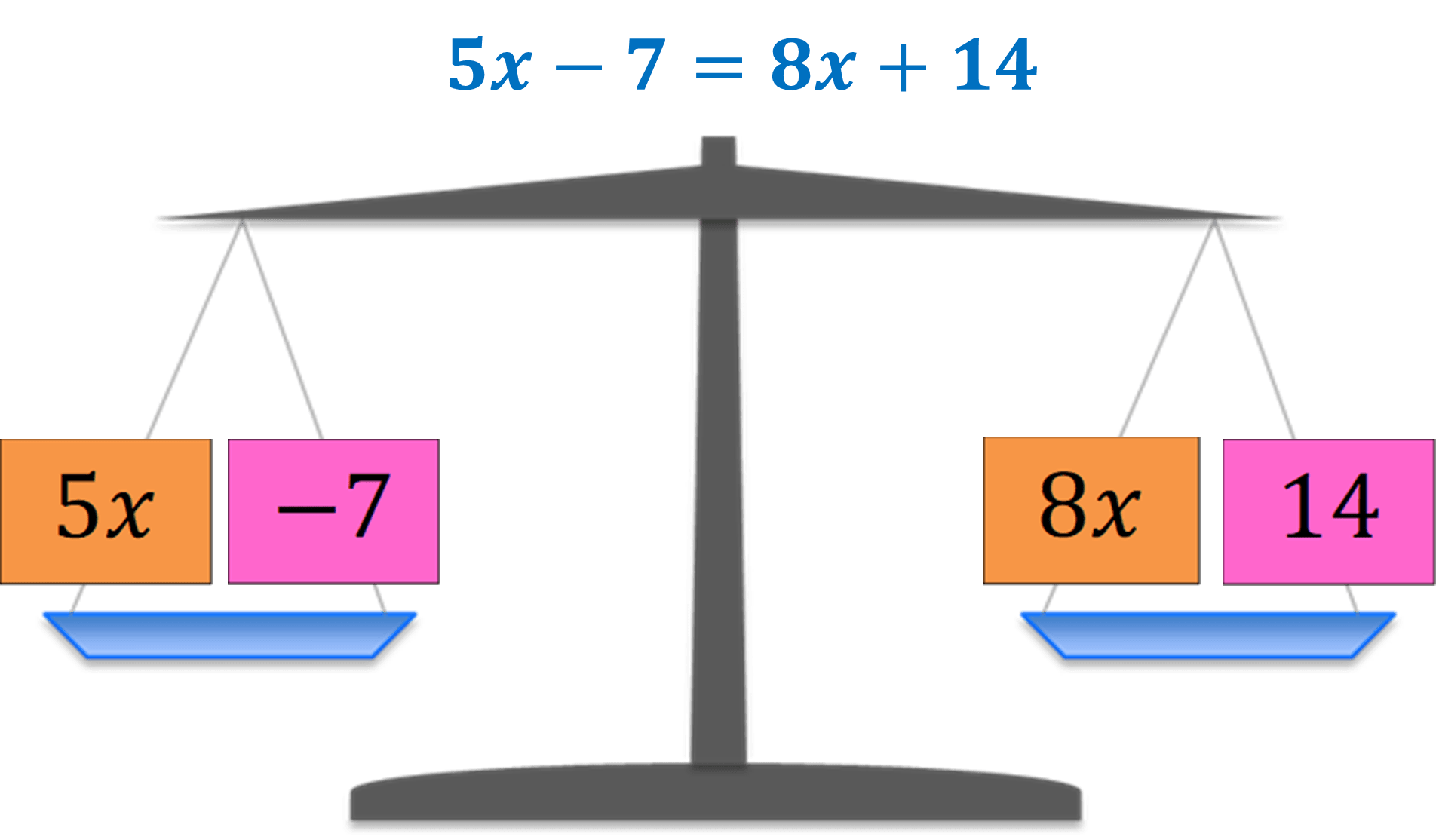• Try moving 8x to the left pan by flipping the post it so that it becomes -8x. Then move -8 to the right pan by flipping the post it so that it becomes 8.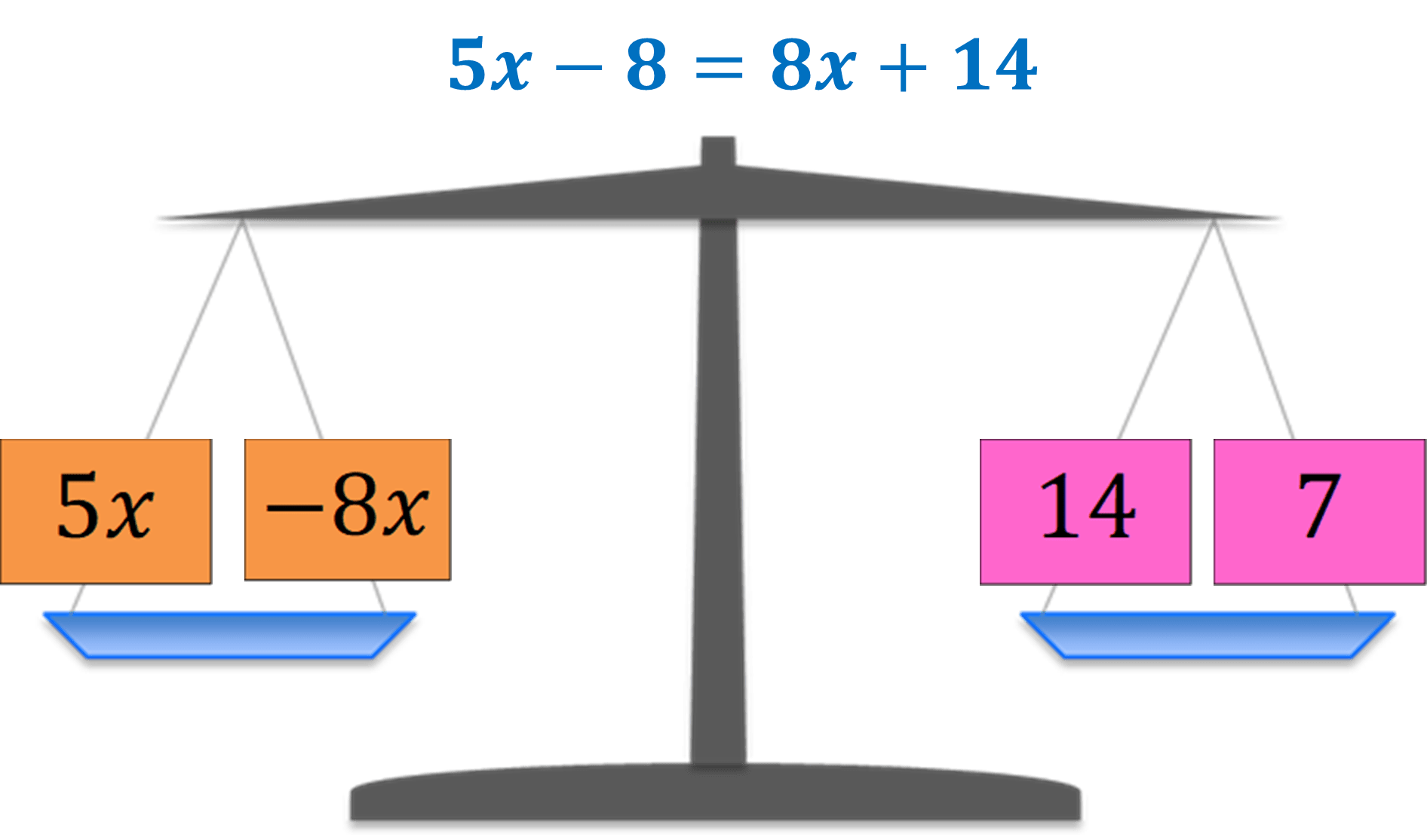• Now it’s easier to solve the equation because all variables are all on the same side, same with the constants.
• Show how it solved:

5x – 8x = 14 + 7

-3x = 21

Divide both sides of the equation by -3.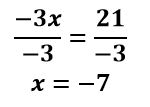• Also, you may try moving 5x to the right instead and let the students figure out if the solution will still be the same.• Show how it solved:

-7 – 14 = 8x – 5

-21 = 3x

Divide both sides of the equation by 3.• Draw out student’s reactions and ask for any clarifications.
• Remind them to check if their solution is correct.

5x – 7 = 8x + 14, x = -7

5(-7) – 7 = 8(-7) + 14

-35 – 7 = -56 + 14

-42 = -42

Therefore, the solution of the equation 5x – 7 = 8x + 14 is -7.

## Extensions

• Have students work in small groups to create their own game that shows how to move variable terms and show how to move variable terms: jeopardy game, card game, bingo game – be sure to include equations and answer sheet.
• Create a movable equation board with student chosen materials, to show what happens when variables and constants move from one side to the other.

### Discover More Math Resources!

If you enjoyed the knowledge and content available here, there are hundreds more lessons available on MathTeacherCoach.com. Take back your time and spend it where you want, not on lesson prep!